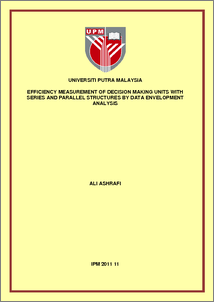# Efficiency Measurement of Decision Making Units with Series and Parallel Structures by Data Envelopment Analysis

## Citation

Ashrafi, Ali (2011) Efficiency Measurement of Decision Making Units with Series and Parallel Structures by Data Envelopment Analysis. Doctoral thesis, Universiti Putra Malaysia.

## Abstract

Data Envelopment Analysis (DEA) is a well-known technique for measuring the efficiency of production processes called decision-making units (DMUs). One of the drawbacks of the standard DEA models is the neglect of internal activities within a DMU. In these models, each DMU is treated as a black box in which the efficiency is assessed by considering the initial inputs and final outputs of the DMU. In this research, the internal structure of DMUs in the DEA model is considered. Knowing about the internal structure of DMUs provides more insights for efficiency evaluation. Because of their basic structures, it is focused on DMUs, whose internal structure is composed of processes connected in series or parallel. Since DEA models in the literature about multi-stage systems have limitations in efficiency measurement, new DEA models are proposed to overcome them. Current multi-stage DEA models utilize the radial measure of efficiency that results in two limitations: firstly, they assume proportional change of inputs or outputs and secondly, the models disregard the existence of slacks in efficiency measurement. Also, in series multi-stage DEA models, the usual method of adjusting the inputs or outputs by the efficiency scores cannot project the inefficient DMUs onto an efficient frontier. To overcome these drawbacks, non-radial DEA models for series and parallel systems in the slacks-based measures (SBM) framework are proposed. These models are suitable for measuring efficiencies when inputs and outputs change nonproportionally and cover all the inefficiencies including input and output slacks. By this model, the DEA frontier points of inefficient DMUs are determined. Furthermore, multi-stage hybrid DEA models in the presence of radial and non-radial inputs/outputs are introduced which integrate both radial and non-radial assessments in a unified manner. The existing DEA models for series systems do not provide the efficiency formulation and linearized transformation if the sharing inputs/outputs exist. In this research, a DEA model is introduced for such systems in which the linearity of the DEA model is preserved. It is significant that unlike the existing DEA models, the proposed model is applicable for both constant returns to scale (CRS) and variable returns to scale (VRS) assumptions. The exact known inputs and outputs are required in the available multi-stage DEA models. However, in the real world, the concern is systems with interval (bounded) data. So, these DEA models do not preserve their linearity. To solve this problem, we proposed an alternative approach that transforms the non-linear DEA model into an equivalent linear model. Also, the interval DEA models are proposed to measure the lower and upper bounds of the efficiency of each DMU with interval data. Furthermore, to compute technical, scale and mix efficiencies of series and parallel systems, DEA models are proposed. Consequently, the local returns to scale (RTS) and the sources of inefficiencies in these systems can be determined. Also, assuming the costs and prices of inputs and outputs are known, the overall cost, allocative, revenue and profit efficiencies of series and parallel systems are computed. Finally, numerical illustrations are provided for each model to explain the proposed models and allow comparison with recent related DEA models.Preview
PDF
IPM_2011_11_ir.pdfView Item